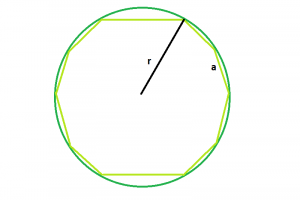# Area of decagon inscribed within the circle

Given here is a regular decagon, inscribed within a circle of radius r, the task is to find the area of the decagon.

Examples:

```Input: r = 5
Output: 160.144

Input: r = 8
Output: 409.969
```Approach:
We know, side of the decagon within the circle, a = r√(2-2cos36)(Refer here)
So, area of the decagon,

A = 5*a^2*(√5+2√5)/2 = 5 *(r√(2-2cos36))^2*(√5+2√5)/2=(5*r^2*(3-√5)*(√5+2√5))/4

Below is the implementation of the above approach:

## C++

 `// C++ Program to find the area of the decagon ` `// inscribed within a circle ` `#include ` `using` `namespace` `std; ` ` `  `// Function to find the area of the decagon ` `float` `area(``float` `r) ` `{ ` ` `  `    ``// radius cannot be negative ` `    ``if` `(r < 0) ` `        ``return` `-1; ` ` `  `    ``// area of the decagon ` `    ``float` `area = (5 * ``pow``(r, 2) * (3 - ``sqrt``(5)) ` `                  ``* (``sqrt``(5) + (2 * ``sqrt``(5)))) ` `                 ``/ 4; ` `    ``return` `area; ` `} ` ` `  `// Driver code ` `int` `main() ` `{ ` `    ``float` `r = 8; ` `    ``cout << area(r) << endl; ` ` `  `    ``return` `0; ` `} `

## Java

 `// Java Program to find the area of the decagon  ` `// inscribed within a circle  ` ` `  `import` `java.io.*; ` ` `  `class` `GFG { ` `     `  `// Function to find the area of the decagon  ` `static` `double` `area(``double`  `r)  ` `{  ` ` `  `    ``// radius cannot be negative  ` `    ``if` `(r < ``0``)  ` `        ``return` `-``1``;  ` ` `  `    ``// area of the decagon  ` `    ``double`  `area = (``5` `* Math.pow(r, ``2``) * (``3` `- Math.sqrt(``5``))  ` `                ``* (Math.sqrt(``5``) + ((``2` `* Math.sqrt(``5``))))/ ``4``);  ` `    ``return` `area;  ` `}  ` ` `  `// Driver code  ` `     `  `    ``public` `static` `void` `main (String[] args) { ` `        ``double`  `r = ``8``;  ` `        ``System.out.println (area(r));  ` `    ``} ` `//This code is contributed by ajit ` `} `

## Python3

 `# Python3 Program to find the area of ` `# the decagon inscribed within a circle ` `from` `math ``import` `sqrt,``pow` ` `  `# Function to find the  ` `# area of the decagon ` `def` `area(r): ` `     `  `    ``# radius cannot be negative ` `    ``if` `r < ``0``: ` `        ``return` `-``1` ` `  `    ``# area of the decagon ` `    ``area ``=` `(``5` `*` `pow``(r, ``2``) ``*` `(``3` `-` `sqrt(``5``)) ``*`  `                 ``(sqrt(``5``) ``+` `(``2` `*` `sqrt(``5``))))``/` `4` `    ``return` `area ` ` `  `# Driver code ` `if` `__name__ ``=``=` `'__main__'``: ` `    ``r ``=` `8` `    ``print``(area(r)) ` ` `  `# This code is contributed ` `# by Surendra_Gangwar `

## C#

 `// C# Program to find the area of the  ` `// decagon inscribed within a circle  ` `using` `System; ` ` `  `class` `GFG ` `{ ` `         `  `// Function to find the area  ` `// of the decagon  ` `static` `double` `area(``double` `r)  ` `{  ` ` `  `    ``// radius cannot be negative  ` `    ``if` `(r < 0)  ` `        ``return` `-1;  ` ` `  `    ``// area of the decagon  ` `    ``double` `area = (5 * Math.Pow(r, 2) *  ` `                  ``(3 - Math.Sqrt(5)) *  ` `                      ``(Math.Sqrt(5) +  ` `                 ``((2 * Math.Sqrt(5))))/ 4);  ` `    ``return` `area;  ` `}  ` ` `  `// Driver code  ` `static` `public` `void` `Main () ` `{ ` `    ``double` `r = 8;  ` `    ``Console.WriteLine (area(r));  ` `} ` `} ` ` `  `// This code is contributed by akt_mit `

## PHP

 ` `

Output:

```409.969
```

My Personal Notes arrow_drop_upCheck out this Author's contributed articles.

If you like GeeksforGeeks and would like to contribute, you can also write an article using contribute.geeksforgeeks.org or mail your article to contribute@geeksforgeeks.org. See your article appearing on the GeeksforGeeks main page and help other Geeks.

Please Improve this article if you find anything incorrect by clicking on the "Improve Article" button below.

Article Tags :
Practice Tags :

Be the First to upvote.

Please write to us at contribute@geeksforgeeks.org to report any issue with the above content.